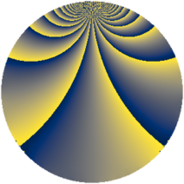# Properties

 Label 2850.2.yLevel $2850$ Weight $2$ Character orbit 2850.y Rep. character $\chi_{2850}(569,\cdot)$ Character field $\Q(\zeta_{10})$ Dimension $800$ Sturm bound $1200$

# Learn more about

## Defining parameters

 Level: $$N$$ $$=$$ $$2850 = 2 \cdot 3 \cdot 5^{2} \cdot 19$$ Weight: $$k$$ $$=$$ $$2$$ Character orbit: $$[\chi]$$ $$=$$ 2850.y (of order $$10$$ and degree $$4$$) Character conductor: $$\operatorname{cond}(\chi)$$ $$=$$ $$1425$$ Character field: $$\Q(\zeta_{10})$$ Sturm bound: $$1200$$

## Dimensions

The following table gives the dimensions of various subspaces of $$M_{2}(2850, [\chi])$$.

Total New Old
Modular forms 2432 800 1632
Cusp forms 2368 800 1568
Eisenstein series 64 0 64

## Trace form

 $$800q + 200q^{4} + O(q^{10})$$ $$800q + 200q^{4} - 200q^{16} + 12q^{19} + 24q^{25} - 4q^{30} - 24q^{39} + 80q^{45} - 768q^{49} + 24q^{54} - 48q^{61} - 40q^{63} + 200q^{64} + 32q^{66} - 80q^{73} + 8q^{76} - 88q^{81} + 104q^{85} + 64q^{99} + O(q^{100})$$

## Decomposition of $$S_{2}^{\mathrm{new}}(2850, [\chi])$$ into newform subspaces

The newforms in this space have not yet been added to the LMFDB.

## Decomposition of $$S_{2}^{\mathrm{old}}(2850, [\chi])$$ into lower level spaces

$$S_{2}^{\mathrm{old}}(2850, [\chi]) \cong$$ $$S_{2}^{\mathrm{new}}(1425, [\chi])$$$$^{\oplus 2}$$